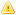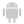Home |Sign in | EnglishHelpWLanguageWLanguage functionsControls, pages and windowsDrawing functionsPrefix syntaxDrawing functions on WDPic variablesDrawPolylinePresentationExampleCoordinatesPattern and PHPDrawing in Browser codeRelated examplesSee alsoOpenSaveAlphaBlendBackgroundChangeModeDrawArcDrawChordDrawCircleDrawLineDrawPointDrawPolygonDrawPolylineDrawRectangleDrawRectangleGradientDrawRoundedRectangleDrawSliceDrawTextDrawTextRTFEndDrawingFillFontHorizontalSymmetryInvertColorMergeLayerPixelColorPixelOpacityResizeRotationSaveStartDrawingVerticalSymmetryPicMergeLayerPicOpenPicResizePicRotationPicSaveThis content has been translated automatically. Click here to view the French version.
• Coordinates
• Pattern and PHP
• Drawing in Browser code
WINDEVWEBDEVWINDEV MobileOthers<WDPic variable>.DrawPolyline (Function)
In french: <Variable WDPic>.DessinePolyligne
Draws an row composed of several segments in an Variable of the WDPic type.
The first coordinate indicates the starting point of the first segment, then each coordinate indicates the end of the previous segment and the beginning of the next segment.
Example
MonImageWDPic is WDPic = "Test.gif"

MonImageWDPic.Pen(LightBlue, 2, 4)
tabCoordonnéesXY2 is array of 0 int = [2, 2, 140, 2, 150, 70, 0, 100, 200, 100]
MonImageWDPic.DrawPolyline(tabCoordonnéesXY2)

IMG_MonDessin = MonImageWDPic
Syntax

Building a line point by point

<WDPic image>.DrawPolyline(<NbPoint> , <X1> , <Y1> , <X2> , <Y2> [, <Xn> [, <Yn> [, <Line color>]]])
<WDPic image>: WDPic variable
Name of the variable of type WDPic to be used. Only the background layer will be handled.
<NbPoint>: Integer
Number of line segments. This parameter defines the number of <X>, <Y> pairs to specify in the function.
<X1>: Integer
X-coordinate of the first segment of the line. These coordinates are expressed in pixels.
<Y1>: Integer
Y-coordinate of the first segment of the line. These coordinates are expressed in pixels.
<X2>: Integer
X-coordinate of the second segment of the line. These coordinates are expressed in pixels.
<Y2>: Integer
Y-coordinate of the second segment of the line. These coordinates are expressed in pixels.
<Xn>: Optional integer
X-coordinate of the N segment of the line. These coordinates are expressed in pixels.
<Yn>: Optional integer
Y-coordinate of the N segment of the line. These coordinates are expressed in pixels.
<Line color>: Integer or constant (optional)
Line color. This color can correspond to:
If this parameter is not specified, the line color:

Building a line via an array of X and Y-coordinates

<WDPic image>.DrawPolyline(<Array of coordinates> [, <Line color>])
<WDPic image>: WDPic variable
Name of the variable of type WDPic to be used. Only the background layer will be handled.
<Array of coordinates>: Array of integers or array of points
WLanguage array of integers containing the coordinates of segments that make up the line. The even subscript represent the X-coordinates while the odd subscripts represent the Y-coordinates of points. These coordinates are expressed in pixels.
<Line color>: Integer or constant (optional)
Line color. This color can correspond to:
If this parameter is not specified, the line color:Versions 26 and laterConstruction of an row via an Variable type Polygon

<WDPic image>.DrawPolyline(<Polygon> [, <Line color>])
<WDPic image>: WDPic variable
Name of the variable of type WDPic to be used. Only the background layer will be handled.
<Polygon>: Variable type Polygon
Name of the variable of type Polygon describing the different points of the Polygon.
<Line color>: Integer or constant (optional)
Line color. This color can correspond to:
If this parameter is not specified, the line color:New in version 26Construction of an row via an Variable type Polygon

<WDPic image>.DrawPolyline(<Polygon> [, <Line color>])
<WDPic image>: WDPic variable
Name of the variable of type WDPic to be used. Only the background layer will be handled.
<Polygon>: Variable type Polygon
Name of the variable of type Polygon describing the different points of the Polygon.
<Line color>: Integer or constant (optional)
Line color. This color can correspond to:
If this parameter is not specified, the line color:Construction of an row via an Variable type Polygon

<WDPic image>.DrawPolyline(<Polygon> [, <Line color>])
<WDPic image>: WDPic variable
Name of the variable of type WDPic to be used. Only the background layer will be handled.
<Polygon>: Variable type Polygon
Name of the variable of type Polygon describing the different points of the Polygon.
<Line color>: Integer or constant (optional)
Line color. This color can correspond to:
If this parameter is not specified, the line color:
Remarks

Coordinates

Coordinates are given with respect to the top left corner of the image (coordinates: (0.0)).
Related Examples:Unit examples (WINDEV): The drawing functions [ + ] Using the main drawing functions of WINDEV to:- Initialize an Image control for drawing- Draw simple shapes- Write a text into a drawing- Change the color in a drawing
Business / UI classification: Neutral code
Component: wd270pnt.dll
Minimum version required
• Version 25